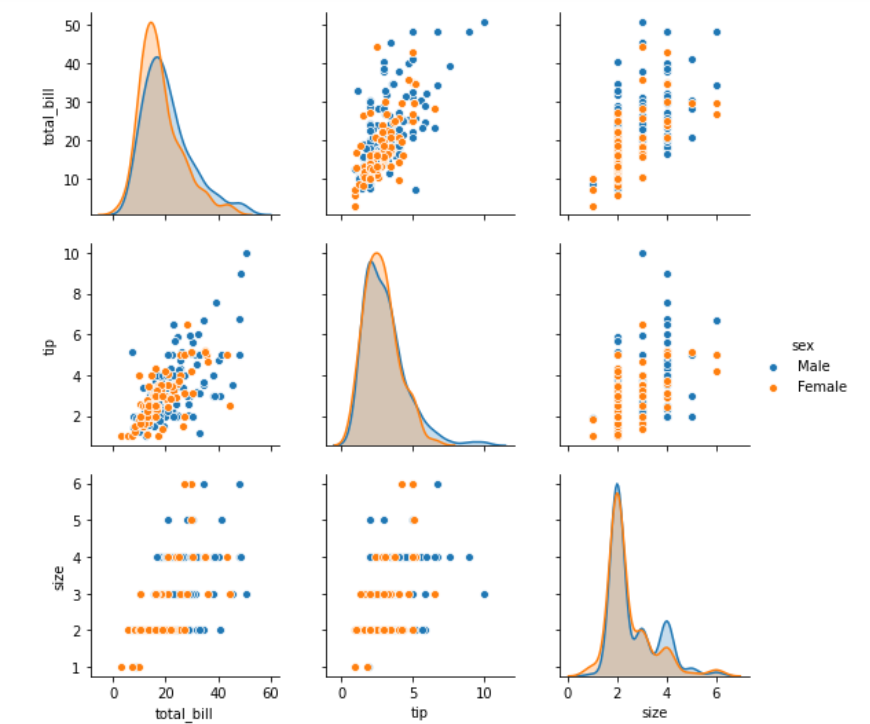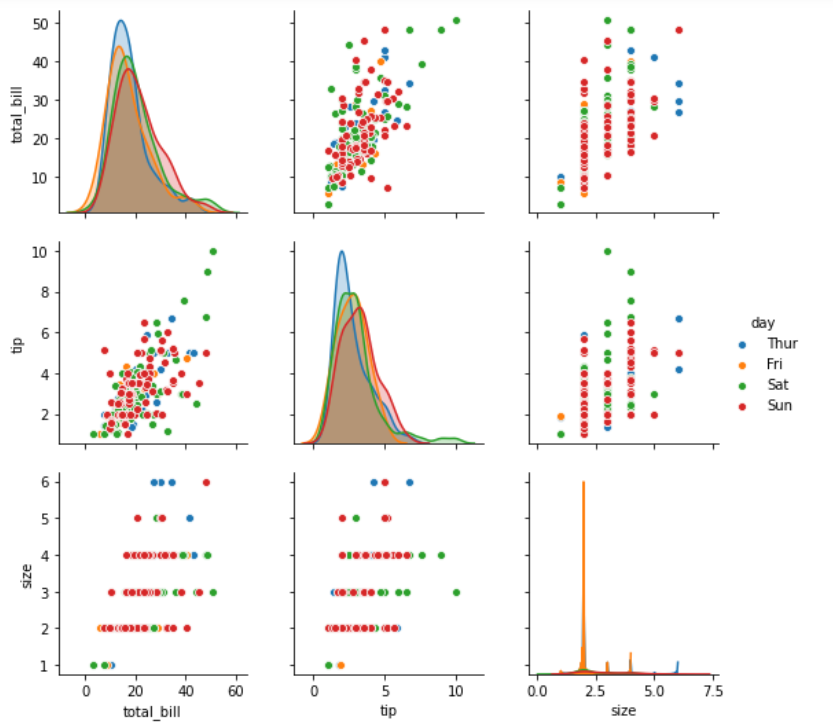Open in App
Not now

# Python – seaborn.pairplot() method

• Difficulty Level : Medium
• Last Updated : 11 Nov, 2022

#### Prerequisite: Seaborn Programming Basics

Seaborn is a Python data visualization library based on matplotlib. It provides a high-level interface for drawing attractive and informative statistical graphics. Seaborn helps resolve the two major issues while working with Matplotlib:

• Default Matplotlib parameters
• Working with data frames

As Seaborn compliments and enhances Matplotlib, the learning curve is quite gradual. If you are familiar with Matplotlib, you are already halfway done with Seaborn.

### seaborn.pairplot() :

To plot multiple pairwise bivariate distributions in a dataset, you can use the .pairplot() function.

The diagonal plots are the univariate plots, and this displays the relationship for the (n, 2) combination of variables in a DataFrame as a matrix of plots.

`                        seaborn.pairplot( data, \*\*kwargs )`

Seaborn.pairplot uses many arguments as input, main of which are described below in form of table:

Below is the implementation of above method:

## Python3

 `# importing packages` `import` `seaborn` `import` `matplotlib.pyplot as plt`   `############# Main Section ############` `# loading dataset using seaborn` `df ``=` `seaborn.load_dataset(``'tips'``)` `# pairplot with hue sex` `seaborn.pairplot(df, hue ``=``'sex'``)` `# to show` `plt.show()`   `# This code is contributed by Deepanshu Rustagi.`

#### Output :## Python3

 `# importing packages` `import` `seaborn` `import` `matplotlib.pyplot as plt`   `############# Main Section ############` `# loading dataset using seaborn` `df ``=` `seaborn.load_dataset(``'tips'``)` `# pairplot with hue day` `seaborn.pairplot(df, hue ``=``'day'``)` `# to show` `plt.show()`   `# This code is contributed by Deepanshu Rustagi.`

#### Output :My Personal Notes arrow_drop_up
Related Articles